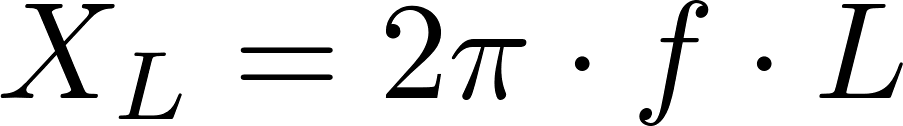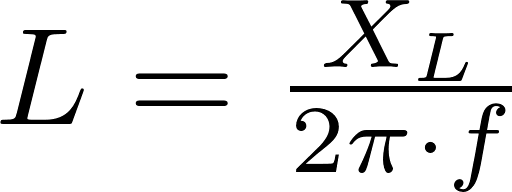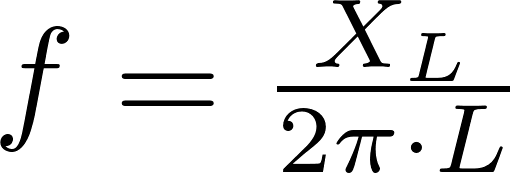# Inductive reactance

Inductive reactance is the opposition to the change of current across an element. The reactance gets larger if the frequency and inductance get larger.

### Formulas

The reactance, inductance and frequency can be calculated with the formulas below.X L is the symbol for inductive reactance and is measured in (Ω).
L is the symbol for inductance and is measured in henry (H).
f is the symbol for frequency and is measured in hertz (Hz).